GCSE Maths Probability

Describing Probability

# Describing Probability

Here we will learn about describing probability, including describing probability using words, recognising the likelihood of an event and placing events on a probability scale.

There are also describing probability worksheets based on Edexcel, AQA and OCR exam questions, along with further guidance on where to go next if you’re still stuck.

## What is describing probability?

Describing probability is when we make judgements as to whether an event will take place. To do this we use words to describe how probable the event is.

For example,

We may say that it is likely to rain tomorrow, or that it is impossible for the sun not to rise.

Some other common words used to describe the probability of an event happening include; certain, very likely, even chance, unlikely and very unlikely.These words can be placed on a probability scale starting at 0 (impossible) and ending at 1 (certain).

### What is describing probability?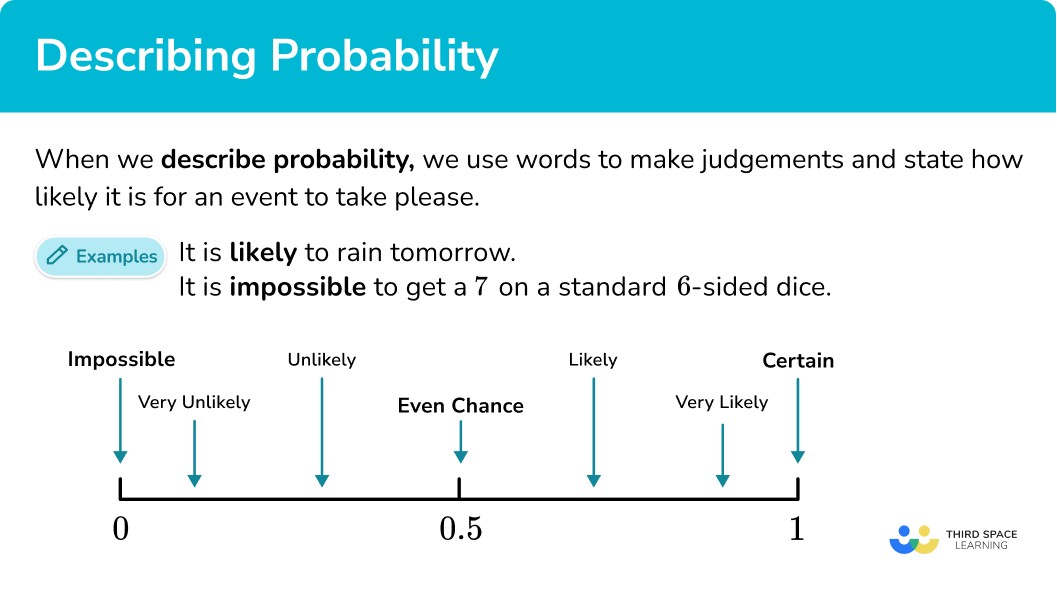### Probability scale

The probability scale is used to represent probabilities on a number line from 0 to 1. In maths, we more often than not use numbers to describe probabilities. Hence, the probability scale starts at 0 (impossible) and ends at 1 (certain).

You could also be asked to place events on a probability scale. To do this you need to consider the words suitable to describe the probability of the event happening, or the number which would represent the chance of the event happening.

For example,

Step-by-step guide: Probability scale

### Probability symbol

Probability symbols are used to write mathematical statements describing probability. These symbols allow us to shorten a mathematical statement to make it more clear and concise. These symbols will also be seen in set notation when using Venn diagrams to represent information.

For example,

Here are the key set notation used in probability, along with their descriptions.

Step-by-step guide: Probability symbol

### Probability notation

In maths we use some common probability notation in addition to standard mathematical notation and symbols. We use set notation as an efficient way of writing the probability of events happening or not happening. Events are notated using capital letters, as well as the use of some greek letters.

For example,

Probability, P(A) refers to the probability that an event, event A in this case, will occur.

Probability, P(B^{\prime}) refers to the probability that an event, event B in this case, will not occur.

Probability, P(A \cup B) refers to the probability of event A or event B happening or both.

Probability, P(A \cap B) refers to the probability of event A and B happening.

Step-by-step guide: Probability notation

## How to describe probability in words

In order to describe probability in words:

1. Consider whether the event you are trying to describe will definitely happen or not happen.
2. Consider the likeliness of the event happening.
3. Choose an appropriate word from the list to describe the probability.

### Explain how to describe probability in words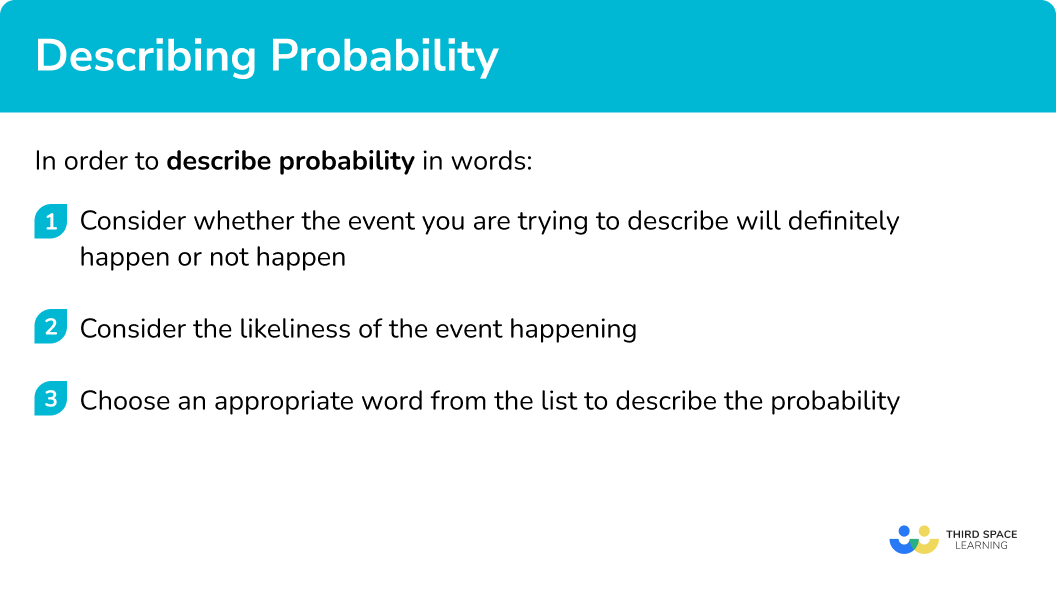## Describing probability in words examples

### Example 1: describing probability in words

Describe the likelihood that a newborn baby is a boy.

1. Consider whether the event you are trying to describe will definitely happen or not happen.

A newborn baby being a boy could happen, so we cannot describe it as impossible

A newborn baby can be a boy or a girl, so we cannot describe it as certain.

2Consider the likeliness of the event happening.

A newborn baby can be a boy or a girl. There are two possible options, both have a 50\% chance of happening.

3Choose an appropriate word from the list to describe the probability.

Two equally likely possible outcomes means we can say that there is an even chance that a newborn baby is a boy.

### Example 2: describing probability in words

Describe the likelihood that a number less than 7 will be scored when an ordinary six-sided dice is rolled once.

Consider whether the event you are trying to describe will definitely happen or not happen.

Consider the likeliness of the event happening.

Choose an appropriate word from the list to describe the probability.

### Example 3: describing probability in words

In words, describe the probability that a red counter is obtained when a counter is taken at random from a bag that contains 11 red counters and 2 blue counters.

Consider whether the event you are trying to describe will definitely happen or not happen.

Consider the likeliness of the event happening.

Choose an appropriate word from the list to describe the probability.

## How to place events on a probability scale

In order to place events on a probability scale:

1. Consider whether the event you are trying to describe will definitely happen or not happen.
2. Consider the likeliness of the event happening.
3. Choose the appropriate place on the scale to place the event.

### Explain how to place events on a probability scale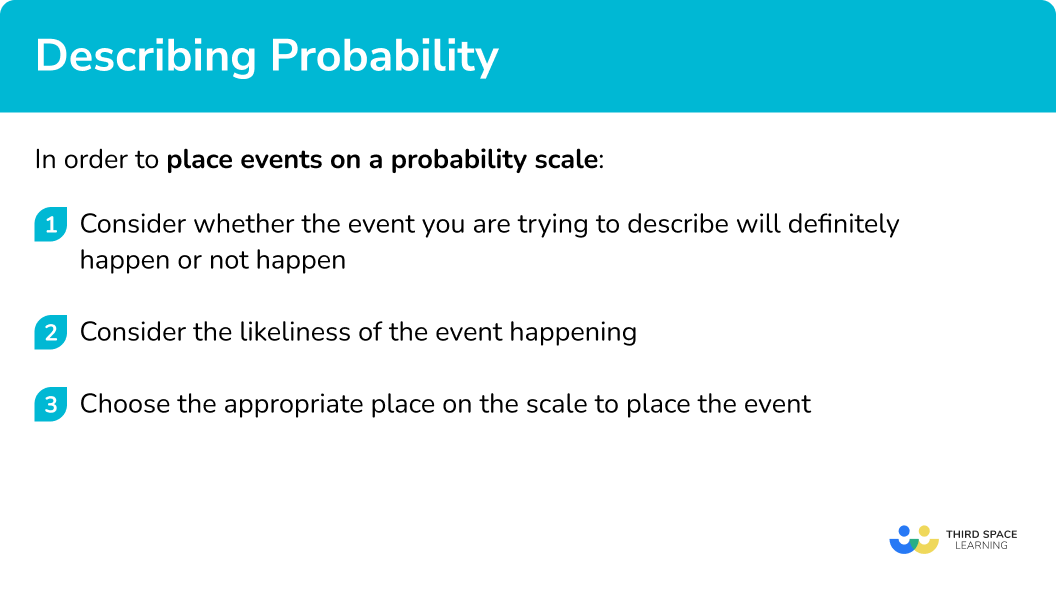## Placing events on a probability scale examples

### Example 4: placing events on a probability scale

Mark on the scale below, the probability that an ordinary six-sided dice is rolled and lands on an even number.

Consider whether the event you are trying to describe will definitely happen or not happen.

Consider the likeliness of the event happening.

Choose the appropriate place on the scale to place the event.

### Example 5: placing events on a probability scale

There are 12 marbles in a bag.

5 marbles are yellow.

4 marbles are green.

2 marbles are red.

Lucas takes a marble at random from the bag.

On the probability scale, mark the probability that Lucas will take a red marble.

Consider whether the event you are trying to describe will definitely happen or not happen.

Consider the likeliness of the event happening.

Choose the appropriate place on the scale to place the event.

### Example 6: placing events on a probability scale

Trevor uses some letter cards to spell the word “SEPTEMBER”.

Trevor takes one of these cards at random.

Mark on the scale below, the probability that Trevor takes a card with a letter A.

Consider whether the event you are trying to describe will definitely happen or not happen.

Consider the likeliness of the event happening.

Choose the appropriate place on the scale to place the event.

### Common misconceptions

• The probability scale goes from \bf{0} (impossible) to \bf{1} (certain)

Remember that impossible is at 0 and certain is at 1, when probability scales are marked numerically rather than with words.

For example,

• Even Chance

An event can only be described as having an “even chance” of occurring if the number of times it could occur is exactly the same as the other possible outcome.

For example,

When flipping a coin, a coin only has two sides. One contains heads, and the other tails. So the coin landing on heads and the coin landing on tails has an equal chance of happening.

But just because an event has two outcomes, it does not mean that the two events have equal probabilities.

For example,

A cube has six faces. One face is red and the other 5 faces are blue. There are 2 outcomes, red and blue. But the probabilities are different.

The probability of getting red is \frac{1}{6} and the probability of getting blue is \frac{5}{6}.

### Practice describing probability questions

1. Place the words in the box onto the probability scale.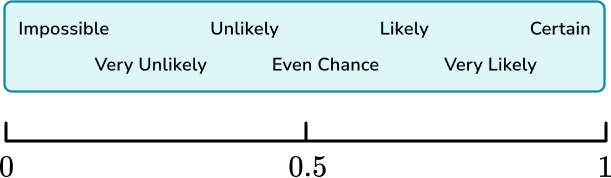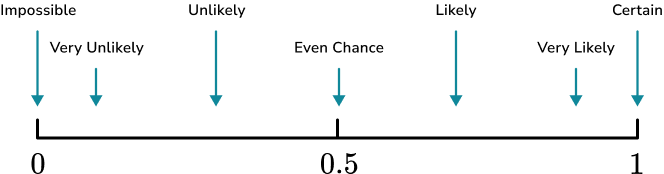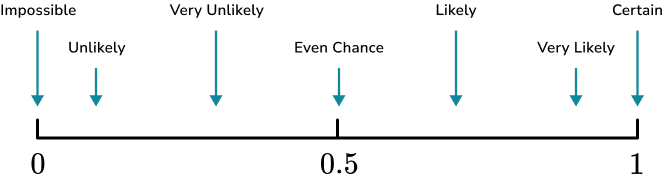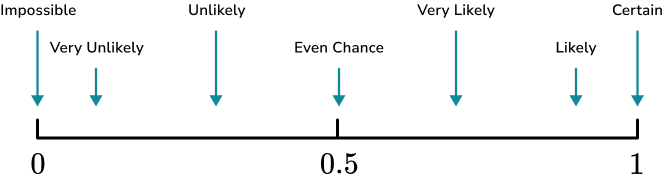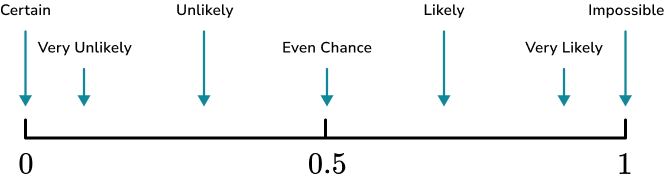Remember that impossible is at 0 and certain is at 1, when probability scales are marked numerically rather than with words.

For example,2. Use a word from the box to describe the probability that a yellow counter is picked from the bag below.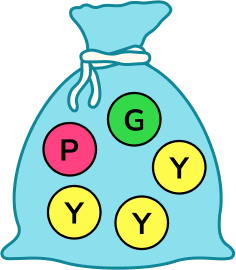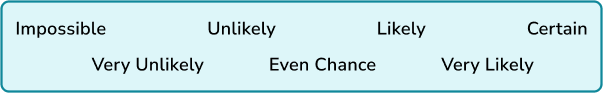ImpossibleUnlikelyCertainVery likelyThere are 3 yellow counters and 2 that are not yellow. There is more chance of picking a yellow counter.

3. Use a word from the box to describe the probability that a green counter is picked from the bag below.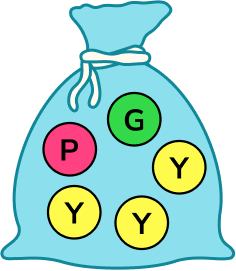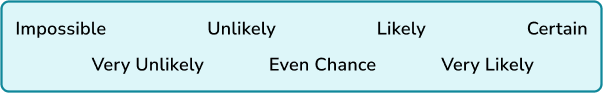LikelyImpossibleVery UnlikelyCertainThere is 1 green counter and 4 that are not green. There is more chance of picking a counter that is not green.

4. Use a word from the box to describe the probability that a red counter is picked from the bag below.UnlikelyImpossibleVery UnlikelyLikelyThere are no red counters in the bag, so a red counter cannot be picked.

5. Caitlin uses some letter cards to spell out the word “DECEMBER”.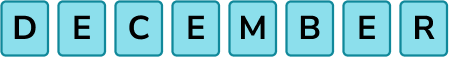Caitlin takes one of these cards at random.

Mark on the scale below, the probability that Caitlin takes a card containing the letter E.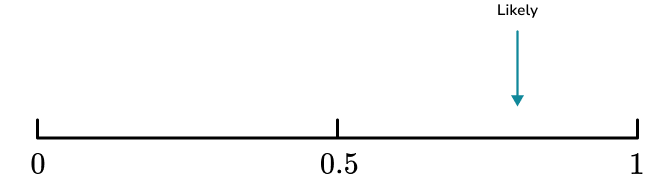There are 3 cards with the letter E and 5 cards that do not contain the letter E. There is more chance of picking a card that does not contain the letter E.

6. Caitlin uses some letter cards to spell out the word “DECEMBER”.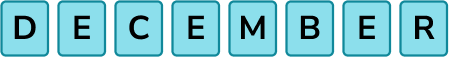Caitlin takes one of these cards at random.

Mark on the scale below, the probability that Caitlin takes a card containing a consonant.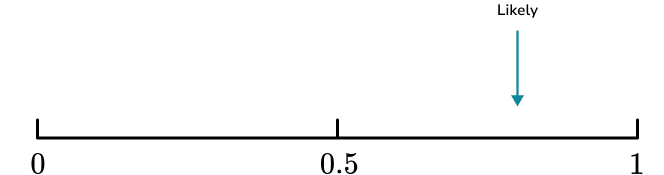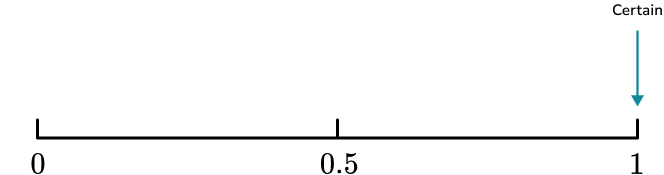There are 5 cards that contain consonants and 3 cards that contain vowels. There is more chance of picking a card that contains a consonant.

### Describing probability GCSE questions

1.Which word from the box best describes the likelihood of each of these events?

(a) A newborn baby is a girl.

(b) You throw an ordinary dice and it lands on a three.

(2 marks)

Even chance

(1)

Unlikely or very unlikely

(1)

2. A fair six-sided dice is thrown.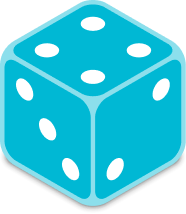The probabilities of the following events have been marked on the probability scale below.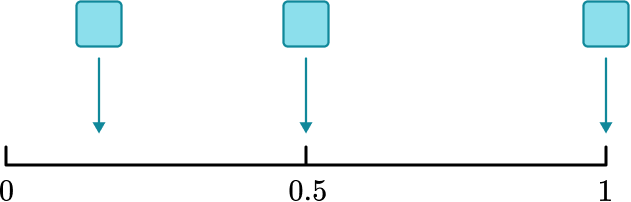A: A number less than 7 is thrown.
B: A “5” is thrown.
C: An odd number is thrown.

Label each place marked on the scale with the appropriate letter.

(3 marks)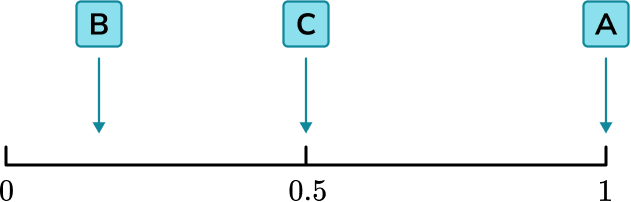One mark per correct letter

(3)

3. Ashton spins a fair spinner with six sections.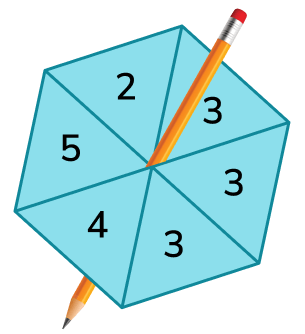Use a word from the box which best describes the probability of each of the following events.

(a) The spinner will land on 3.

(b) The spinner will land on 6.

(c) The spinner will land on 5.

(d) The spinner will land on a number greater than 1.

(4 marks)

(a) Even chance

(1)

(b) Impossible

(1)

(c) Unlikely or very unlikely

(1)

(d) Certain

(1)

## Learning checklist

You have now learned how to:

• Describe probability using words
• Place events on a probability scale
• Reason about the likelihood of an event happening

## Still stuck?

Prepare your KS4 students for maths GCSEs success with Third Space Learning. Weekly online one to one GCSE maths revision lessons delivered by expert maths tutors.

Find out more about our GCSE maths tuition programme.